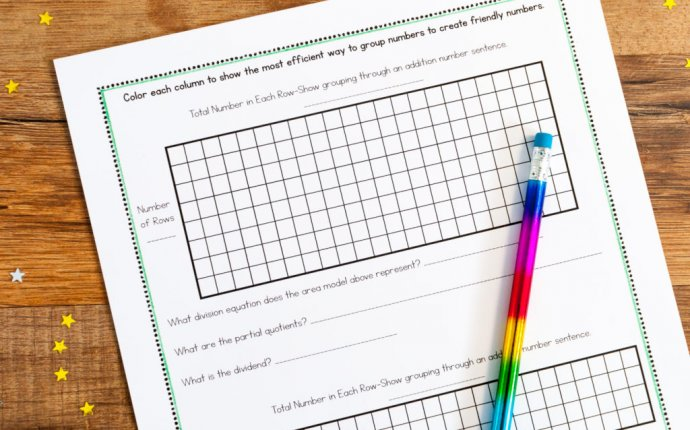strategies for teaching long division

Teaching Strategies / May 24, 2017

4 does not go into 1 (hundred). So combine the 1 hundred with the 6 tens (160).

4 goes into 16 four times.

4 goes into 5 once, leaving a remainder of 1.

 th h t o 0 4 0 0 R7 3 2 0 7

8 does not go into 3 of the thousands. So combine the 3 thousands with the 2 hundreds (3, 200).

8 goes into 32 four times (3, 200 ÷ 8 = 400)
8 goes into 0 zero times (tens).
8 goes into 7 zero times, and leaves a remainder of 7.

Next, students learn to find the remainder using the process of "multiply & subtract". This is a very important step! The "multiply & subtract" part is often very confusing to students, so here we practice it in the easiest possible place: in the very end of the division, in the ones colum (instead of in the tens or hundreds column). Of course, this assumes that students have already learned to find the remainder in easy division problems that are based on the multiplication tables (such as 45 ÷ 7 or 18 ÷ 5).

In the problems before, you just wrote down the remainder of the ones. Usually, we write down the subtraction that actually finds the remainder. Look carefully:

 h t o 0 6 1 2 4 7 − 4

When dividing the ones, 4 goes into 7 one time. Multiply 1 × 4 = 4, write that four under the 7, and subract. This finds us the remainder of 3.

Check: 4 × 61 + 3 = 247

 0 4 0 2 1 6 0 9 − 8

When dividing the ones, 4 goes into 9 two times. Multiply 2 × 4 = 8, write that eight under the 9, and subract. This finds us the remainder of 1.

Check: 4 × 402 + 1 = 1, 609

Here are some example problems. Now, the students check the answer by multiplying the divisor times the quotient, and then adding the remainder.

 a. b. c. d.

Step 3: A remainder in the tens

In this step, students practice for the first time all the basic steps of long division algorithm: divide, multiply & subtract, drop down the next digit. We use two-digit numbers to keep it simple. Multiply & subtract has to do with finding the remainder, and after finding a remainder, we combine that with the next unit we are getting ready to divide (dropping down the digit).

An example:

1. Divide. 2. Multiply & subtract. 3. Drop down the next digit.

Two goes into 5 two times, or 5 tens ÷ 2 = 2 whole tens - but there is a remainder!

 t o 5 8

To find it, multiply 2 × 2 = 4, write that 4 under the five, and subtract to find the remainder of 1 ten.

 2 9 4 ↓ 1 8

Next, drop down the 8 of the ones next to the leftover 1 ten. You combine the remainder ten with 8 ones, and get 18.

Divide 2 into 18. Place 9 into the quotient.

Multiply 9 × 2 = 18, write that 18 under the 18, and subtract.

The division is over since there are no more digits in the dividend. The quotient is 29.

Step 4: A remainder in any of the place values

After the previous step has been mastered, students then practice long division with three- and four-digit numbers where they will have to go through the basic steps several times.
 2 7 8

Two goes into 2 one time, or 2 hundreds ÷ 2 = 1 hundred.

Multiply 1 × 2 = 2, write that 2 under the two, and subtract to find the remainder of zero.

 1 8 2 ↓ 0 7

Next, drop down the 7 of the tens next to the zero.

Divide. Multiply & subtract. Drop down the next digit.
 1 3

Divide 2 into 7. Place 3 into the quotient.

Multiply 3 × 2 = 6, write that 6 under the 7, and subtract to find the remainder of 1 ten.

 1 8

Next, drop down the 8 of the ones next to the 1 leftover ten.

 1 3 9
 - 1 8

Multiply 9 × 2 = 18, write that 18 under the 18, and subtract to find the remainder of zero.

There are no more digits to drop down. The quotient is 139.

These ideas are also explained in the YouTube video below:

Why long division works

I feel the long division algorithm AND why it works presents quite a complex thing for students to learn, so in this case I don't see a problem with students first learning the algorithmic steps (the "how"), and later delving into the "why". Trying to do both simultaneously may prove to be too much to some.

However, once the student has a basic mastery of how to do long division, it is time to also study what it is based on. To learn more about that, please see:

Worksheets

Long division worksheets
Create an unlimited supply of worksheets for long division (grades 4-6), including with 2-digit and 3-digit divisors. The worksheets can be made in html or PDF format - both are easy to print. You can also customize them using the generator.

Source: www.homeschoolmath.net